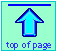Note: this document will print in an appropriately modified format (11 pages)

A chemical equation expresses the net change in composition associated with a chemical reaction by showing the number of moles of reactants and products. But because each component has its own molar mass, equations also implicitly define the way in which the masses of products and reactants are related. In this unit we will concentrate on understanding and making use of these mass relations.

1  How to read and write chemical equations

In a chemical reaction, one or more reactants are transformed into products:

reactants → products

The purpose of a chemical equation is to express this relation in terms of the formulas of the actual reactants and products that define a particular chemical change. For example, the reaction of mercury with oxygen to produce mercuric oxide would be expressed by the equation

Hg + O2 → HgO2

Sometimes, for convenience, it is desirable to indicate the physical state (gas, liquid or solid) of one or more of the species by appropriate abbreviations:

Hg(l) + O2(g) → HgO2(s)

C(graphite) + O2(g) → CO2(g)

C(diamond) + O2(g) → CO2(g)

However, this is always optional.

### Chemical equations must be balanced!

Basically, this means that chemical equations must be consistent with the law of conservation of mass: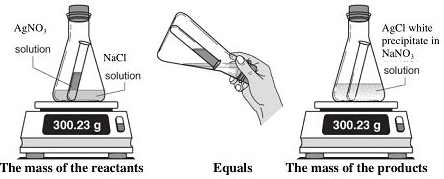In the context of an ordinary chemical reaction, conservation of mass means that atoms are neither created nor distroyed. This requirement is easily met by making sure that there are equal numbers of all atoms on both sides of the equation.

When we balance an equation, we simply make it consistent with the observed fact that individual atoms are conserved in chemical changes.

It often happens, however, that we do end up with a fractional coefficient, as in this variant of the above example.

2  Net ionic equations

Ionic compounds are usually dissociated in aqueous solution; thus if we combine solutions of silver nitrate AgNO3 and sodium chloride NaCl we are really combining four different species: the cations (positive ions) Ag+ and Na+and the anions (negative ions) NO3 and Cl. It happens that when the ions Ag+ and Cl are brought together, they will combine to form an insoluble precipitate of silver chloride. The net equation for this reaction is

Ag+(aq) + Cl(aq)→ AgCl(aq)

Note that

• the ions NO3 and Cl are not directly involved in this reaction; the equation expresses only the net change, which is the removal of the silver and chloride ions from the solution to form an insoluble solid.
• the symbol (aq) signifies that the ions are in aqueous solution, and thus are hydrated, or attached to water molecules.
• the symbol (s) indicates that the substance AgCl exists as a solid. When a solid is formed in a reaction that takes place in solution, it is known as a precipitate. The formation of a precipitate is often indicated by underscoring.

#### Predicting the outcome when dissolved salts are mixed

From the above example involving silver chloride, it is clear that a meaningful net ionic equation can be written only if two ions combine to form an insoluble compound. In order to make this determination, it helps to know the solubility rules— which all students of chemistry were at one time required to memorize, but are nowadays usually obtained from tables such as the one shown below.

3  Mass relations in chemical equations

### Basic "chemical arithmetic"

A balanced chemical equation expresses the relative number of moles of each component (product or reactant), but because each formula in the equation implies a definite mass of the substance (its molar mass), the equation also implies that certain weight relations exist between the components. For example, the equation describing the combustion of carbon monoxide to carbon dioxide

2 CO + O2 → 2 CO2

implies the following relations: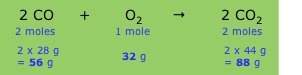The relative masses shown in the bottom line establish the stoichiometry of the reaction, that is, the relations between the masses of the various components. Since these masses vary in direct proportion to one another, we can define what amounts to a conversion factor (sometimes referred to as a chemical factor) that relates the mass of any one component to that of any other component.

But the same relation applies to any common set of mass or weight units, so we can simply say that the mass ratio of CO2-to-CO expressed by the above equation is 1.57, which is also just the mass ratio of these two components in the equation. This, in turn, allows us to easily handle such problems as the following:

### Mastering mass-mass problems

Don't expect to pass Chemistry unless you can handle problems such as the ones below; they come up frequently in all kinds of contexts.  If you feel the need for more guidance, see one of the video tutorials listed near the bottom of this page.

4  Understanding limiting reactants

Most chemical reactions that take place in the real world begin with more or less arbitrary amounts of the various reactants; we usually have to make a special effort if we want to ensure that stoichiometric amounts of the reactants are combined. This means that one or more reactant will usually be present in excess; there will be more present than can react, and some will remain after the reaction is over. At the same time, one reactant will be completely used up; we call this the limiting reactant because the amount of this substance present will control, or limit, the quantities of the other reactants that are consumed as well as the amounts of products produced.

Limiting reactant problems are handled in the same way as ordinary stoichiometry problems with one additional preliminary step: you must first determine which of the reactants is limiting— that is, which one will be completely used up. To start you off, consider the following very simple example

### Limiting reactants in everyday life

The concept of limiting reactants touches us all in our everyday lives — and as we will show in the second example below, even in the maintenance of life itself!

#### Air-to-fuel ratios in combustion

Combustion is an exothermic process in which a fuel is combined with oxygen; complete combustion of a hydrocarbon fuel such as methane or gasoline yields carbon dioxide and water:

CH4 + 2 O2 → CO2 + 2 H2O(g)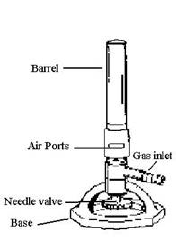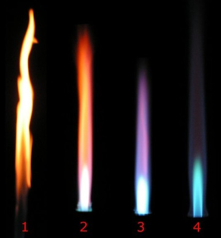Complete combustion of each kg of methane consumes 4 kg of dioxygen, which is supplied by the air. In the classic Bunsen burner, this air is admitted through an adjustable opening near the base. When it is fully open, the flame is blue in color and achieves its maximum temperature, indicating that combustion is approximately stoichiometric. If the opening is gradually closed, the appearance of the flame changes as illustrated. Under these conditions, oxygen becomes the limiting reactant and combustion is incomplete.

Incomplete combustion is generally undesirable because it wastes fuel, produces less heat, and releases pollutants such as carbon soot. Energy-producing combustion processes should always operate in fuel-limited mode.

In ordinary combustion processes, the source of oxygen is air. Because only about 20 percent of the molecules in dry air consist of O2, the volume of air that must be supplied is five times greater than what would be required for pure O2. Calculation of the air-to-fuel mass ratio ("A/F ratio") employed by combustion engineers is complicated by the differing molar masses of dioxygen and air. For methane combustion, the A/F ratio works out to about 17.2. (For the detailed calculation, see here). A/F ratios which exceed the stoichiometric values are said to be lean, while those in which air becomes the limiting component are characterized as rich. In order to ensure complete combustion, it is common practice to maintain a slightly lean mixture. The quantities of so-called excess air commonly admitted to burners vary from 5-10% for natural gas to up to 100% for certain grades of coal.

For internal combustion engines fueled by gasoline (roughly equivalent to
C7H14), the stoichiometric A/F ratio is 15:1. However, practical considerations necessitate differing ratios at various stages of operation. Typical values vary from a rich ratio for starting or acceleration to slightly lean ratios for ordinary driving. These ratios are set by the carburetor, with additional control by the engine computer and exhaust-line oxygen sensor in modern vehicles, or by a manual choke in earlier ones.

#### Aerobic and anaerobic respiration

Our bodies require a continual supply of energy in order to maintain neural activity, synthesize proteins and other essential biochemical components, replace cells, and to power muscular action. The "fuel" — the carrier of chemical energy — glucose, a simple sugar which is released as needed from the starch-like polymer glycogen, the form in which the energy we derive from food is stored.

Arterial blood carries dissolved glucose along with hemoglobin-bound dioxygen to individual cells which are the sites of glucose "combustion":

C6H12O6 + 6 O2 → 6 CO2 + 6 H2O

The net reaction and the quantity of energy released are the same as if the glucose were burned in the open air, but within the cells the reaction proceeds in a series of tiny steps which capture most of this energy for the body's use, liberating only a small fraction of it as thermal energy (heat).

Because this process utilizes oxygen from the air we breath, it is known as aerobic respiration. And as with any efficient combustion process, glucose is the limiting reactant here.

But there are times when vigorous physical activity causes muscles to consume glucose at a rate that exceeds the capacity of the blood to deliver the required quantity of oxygen. Under these conditions, cellular respiration shifts to an alternative anaerobic mode:

C6H12O6 → 2 CH3CH(OH)COOH

As you can see from this equation, glucose is only partially broken down (into lactic acid), and thus only part of its chemical energy is captured by the body.There are numerous health benefits to aerobic exercise, including increased ability of the body to maintain an aerobic condition. But if you are into short-distance running (sprinting) or being pursued by a tiger, the reduced efficiency of anaerobic exercise may be a small price to pay.